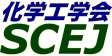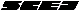## \$B9V1i%W%m%0%i%`!J2q>l!&F|DxJL!K(B

### P\$B2q>l(B \$BBh(B2\$BF|(B

\$B:G=*99?7F|;~!'(B2012-08-20 15:02:17
\$B9V1i(B
\$B;~9o(B
\$B9V1i(B
\$BHV9f(B
\$B9V1iBjL\!?H/I=\$B%-!<%o!<%I(B\$BJ,N`(B
\$BHV9f(B
\$B\$BHV9f(B
\$B%7%s%]%8%&%`(B \$B!c4D6-It2q%7%s%]%8%&%`!d(B
(10:00\$B!A(B11:00)\$B!!(B(\$B:BD9(B \$BK\6?(B \$B>H5W(B)
10:00\$B!A(B 10:20P204\$B433cB\$@.\$KMQ\$\$\$k3\$:=BeBX:`\$H\$7\$F\$N@=9]%9%i%0\$NI>2A(B
(\$B9-Bg1!9)(B) \$B!{(B(\$B@5(B)\$BCf0f(B \$BCR;J(B \$B!&(B \$BCfB<(B \$B>-;V(B \$B!&(B (\$B9-Bg4D0B%;(B) \$B@u2,(B \$BAo(B \$B!&(B (\$B@5(B)\$B1|ED(B \$BE/;N(B \$B!&(B (\$B@5(B)\$B@>Eh(B \$B>D(B \$B!&(B (\$B?e;:Am8&%;(B) \$B:j;3(B \$B0l9'(B \$B!&(B (\$BBgJ,8)G@?e8&%;(B) \$BJFED(B \$B0l5*(B
Clam
larval settlement
steel manufacturing slag
S-10757
10:20\$B!A(B 10:40P205\$BE49]%9%i%0\$+\$i\$NAX>uJ#?e;@2=J*\$N9g@.\$K4X\$9\$k4pAC8&5f(B
(\$B4X@>Bg4D6-ET;T9)(B) \$B!{(B(\$B3X(B)\$B86(B \$BNIB@(B \$B!&(B (\$B3X(B)\$B;09%(B \$B5.G7(B \$B!&(B (\$B@5(B)\$BB<;3(B \$B7{90(B \$B!&(B (\$B@5(B)\$B \$B!&(B (JFE\$B%_%M%i%k(B) \$B1'ED@n(B \$B1YO:(B
iron and steel slag
layered double hydroxide
anion exchanger
S-10403
10:40\$B!A(B 11:00P206\$B%[%?%F3-3L\$r%+%k%7%&%`8;\$H\$7\$?%j%s\$H%U%CAG\$NF1;~=|5n5sF0(B
(\$BKL8+9)Bg(B) \$B!{(B(\$B3X(B)\$B90Cf(B \$B5.G7(B \$B!&(B (\$B@5(B)\$B?{Ln(B \$B5|(B \$B!&(B (\$B@5(B)\$BKYFb(B \$B=_0l(B
Apatite
Removal of phosphorus and fluoride
Scallop shell
S-10264

\$B9V1i%W%m%0%i%`(B
\$B2=3X9)3X2q(B \$BBh(B44\$B2s=)5(Bg2q(B(C) 2012 \$B8x1W
Most recent update: 2012-08-20 15:02:17
For more information contact \$B2=3X9)3X2qElKL;YIt(B \$BBh(B44\$B2s=)5(Bg2q(B \$BLd\$\$9g\$;78(B
E-mail: inquiry-44fwww3.scej.org
This page was generated byeasp 2.28; proghtml 2.28c (C)1999-2011 kawase